Most Affordable JEE | NEET | 8,9,10 Preparation by Kota's Top IITian Doctor Faculties

# Area Under The Curve - JEE Main Previous Year Question with SolutionsJEE Main Previous Year Question of Math with Solutions are available at eSaral. Practicing JEE Main Previous Year Papers Questions of mathematics will help the JEE aspirants in realizing the question pattern as well as help in analyzing weak & strong areas. Get detailed Class 11th &12th Physics Notes to prepare for Boards as well as competitive exams like IIT JEE, NEET etc. eSaral helps the students in clearing and understanding each topic in a better way. eSaral is providing complete chapter-wise notes of Class 11th and 12th both for all subjects. Besides this, eSaral also offers NCERT Solutions, Previous year questions for JEE Main and Advance, Practice questions, Test Series for JEE Main, JEE Advanced and NEET, Important questions of Physics, Chemistry, Math, and Biology and many more. Download eSaral app for free study material and video tutorials.
Q. The area of the region bounded by the parabola $(\mathrm{y}-2)^{2}$ = x – 1, the tangent to the parabola at the point (2, 3) and the x–axis is :- (1) 9            (2) 12            (3) 3              (4) 6 [AIEEE-2009]
Ans. (1)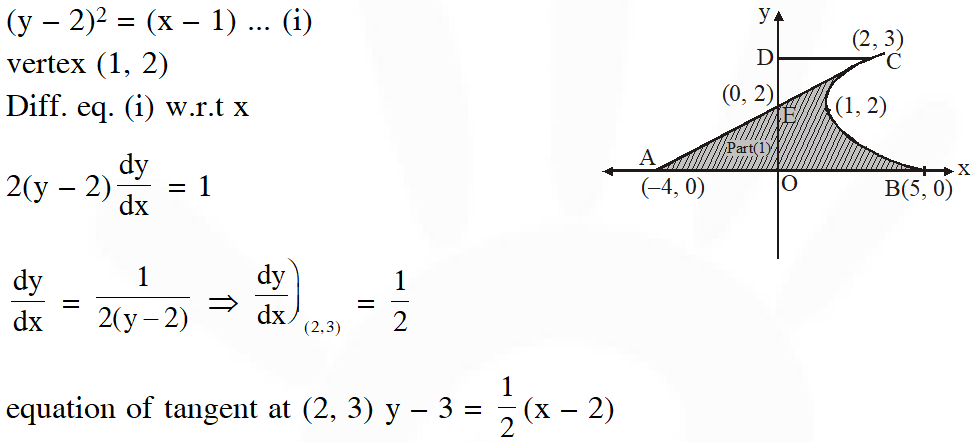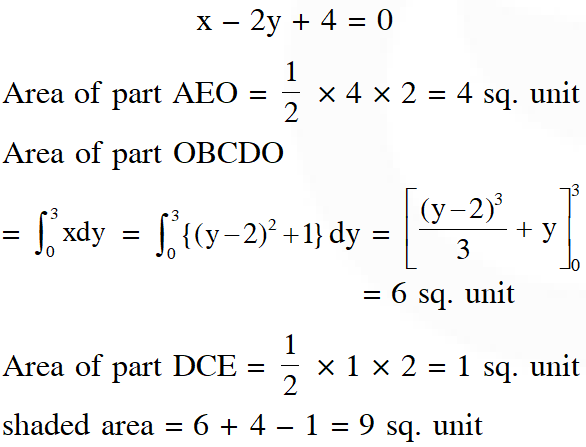Q. The area bounded by the curves y = cos x and y = sin x between the ordinates x = 0 and x = $\frac{3 \pi}{2}$ is :- (1) $4 \sqrt{2}-2$ (2) $4 \sqrt{2}+2$ (3) $4 \sqrt{2}-1$ (4) $4 \sqrt{2}+1$ [AIEEE-2010]
Ans. (1)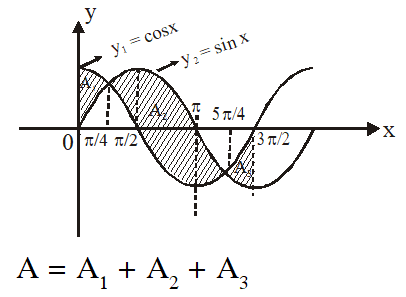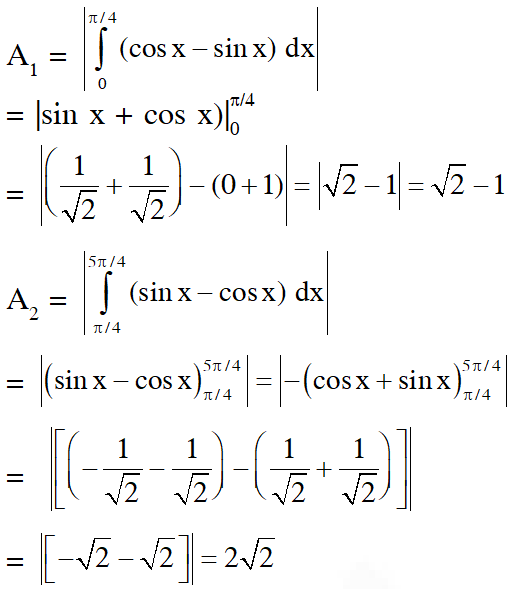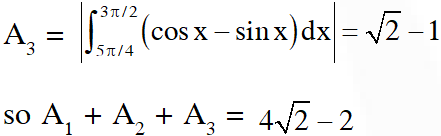Q. The area of the region enclosed by the curves $y=x, x=e, y=\frac{1}{x}$ and the positive $x$ -axis is:- (1) $\frac{3}{2}$ square units (2) $\frac{5}{2}$ square units (3) $\frac{1}{2}$ square units (4) 1 square units [AIEEE-2011]
Ans. (1)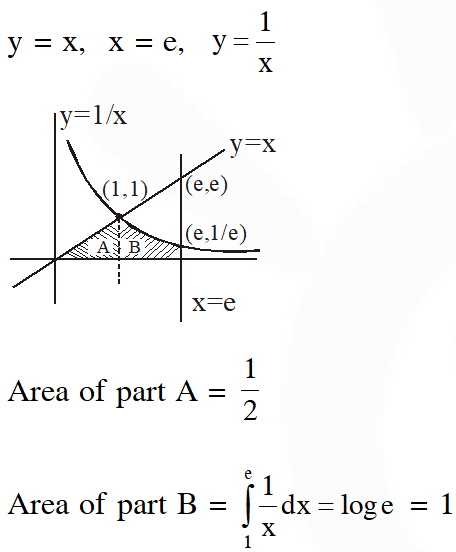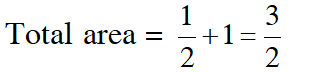Q. The area bounded by the curves $y^{2}=4 x$ and $x^{2}=4 y$ is :- (1) 0             (2) $\frac{32}{3}$              (3) $\frac{16}{3}$               (4) $\frac{8}{3}$ [AIEEE-2011]
Ans. (3)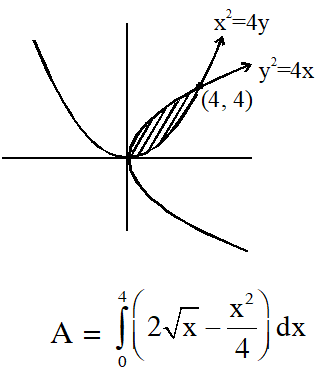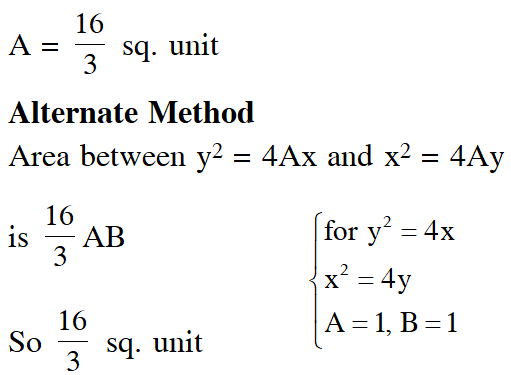Q. The area bounded between the parabolas $x^{2}=\frac{y}{4}$ and $x^{2}=9 y,$ and the straight line $y=2$ is : (1) $10 \sqrt{2}$ (2) $20 \sqrt{2}$ (3) $\frac{10 \sqrt{2}}{3}$ (4) $\frac{20 \sqrt{2}}{3}$ [AIEEE-2012]
Ans. (4)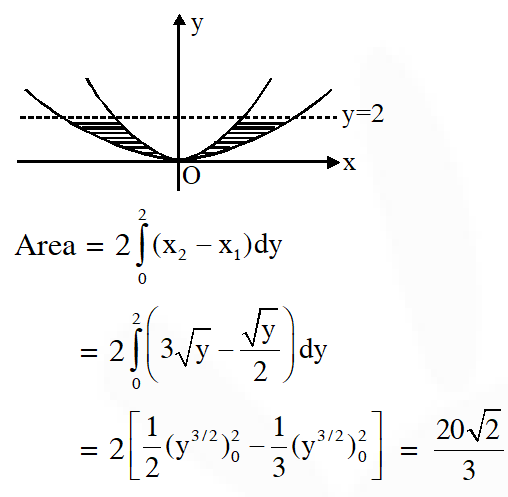Q. The area (in square units) bounded by the curves $y=\sqrt{x}, 2 y-x+3=0,$ x-axis and lying in the first quadrant is : (1) 9         (2) 36            (3) 18           (4) $\frac{27}{4}$ [JEE (Main)-2013]
Ans. (1)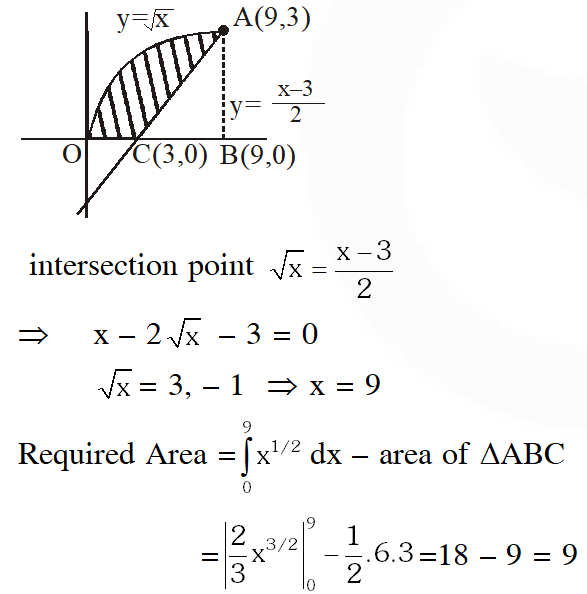Q. The area bounded by the curve y = ln(x) and the lines y = 0, y = ln (3) and x = 0 is equal to : (1) 3 ln (3) – 2                      (2) 3                    (3) 2                      (4) 3 ln (3) + 2 [JEE-Main (On line)-2013]
Ans. (3)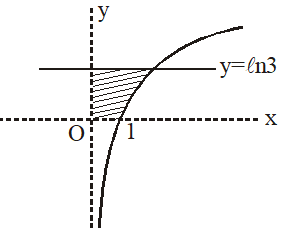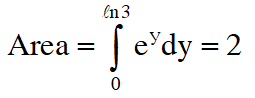Q. The area of the region (in sq. units), in the first quadrant, bounded by the parabola y = $9 x^{2}$ and the lines x = 0, y = 1 and y = 4, is :- (1) 7/9             (2) 14/3              (3) 14/9              (4) 7/3 [JEE-Main (On line)-2013]
Ans. (3)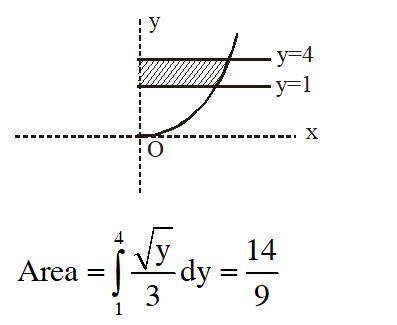Q. The area under the curve $y=|\cos x-\sin x|, 0 \leq x \leq \frac{\pi}{2},$ and above $x$ -axis is : (1) $2 \sqrt{2}$ (2) $2 \sqrt{2}+2$ (3) 0 (4) $2 \sqrt{2}-2$ [JEE-Main (On line)-2013]
Ans. (4) Area $=2 \int_{0}^{\pi / 4}(\cos x-\sin x) d x=-2+2 \sqrt{2}$
Q. Let $f:[-2,3] \rightarrow[0, \infty)$ be a continuous function such that $f(1-x)=f(x)$ for all $x \in[-2,3] .$ If $R_{1}$ is the numerical value of the area of the region bounded by $y=f(x), x=-2, x=3$ and the axis of $x$ and $R_{2}=\int_{-2}^{3} x f(x) d x,$ then : (1) $2 \mathrm{R}_{1}=3 \mathrm{R}_{2}$ (2) $\mathrm{R}_{1}=\mathrm{R}_{2}$ (3) $3 \mathrm{R}_{1}=2 \mathrm{R}_{2}$ (4) $\mathrm{R}_{1}=2 \mathrm{R}_{2}$ [JEE-Main (On line)-2013]
Ans. (4)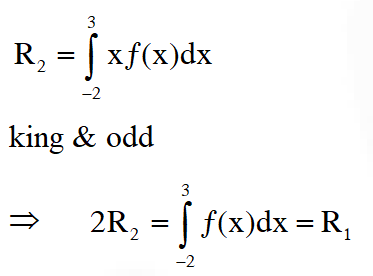Q. The area of the region described by $\mathrm{A}=\left\{(\mathrm{x}, \mathrm{y}): \mathrm{x}^{2}+\mathrm{y}^{2} \leq 1 \text { and } \mathrm{y}^{2} \leq 1-\mathrm{x}\right\}$ is : (1) $\frac{\pi}{2}+\frac{4}{3}$ (2) $\frac{\pi}{2}-\frac{4}{3}$ (3) $\frac{\pi}{2}-\frac{2}{3}$ (4) $\frac{\pi}{2}+\frac{2}{3}$ [JEE(Main)-2014]
Ans. (1)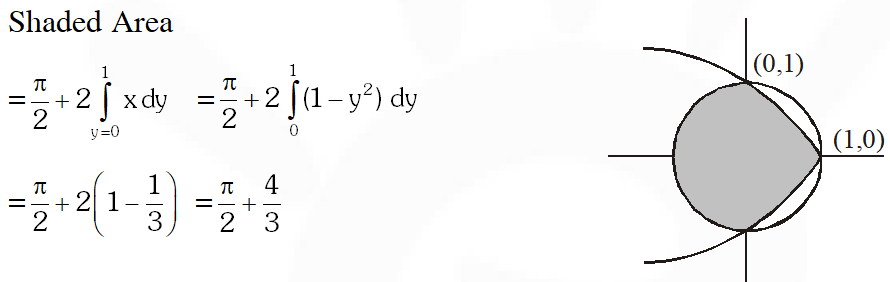Q. The area (in sq.units) of the region $\left\{(\mathrm{x}, \mathrm{y}): \mathrm{y}^{2} \geq 2 \mathrm{x} \text { and } \mathrm{x}^{2}+\mathrm{y}^{2} \leq 4 \mathrm{x}, \mathrm{x} \geq 0, \mathrm{y} \geq 0\right\}$ is :- (1) $\frac{\pi}{2}-\frac{2 \sqrt{2}}{3}$ (2) $\pi-\frac{4}{3}$ (3) $\pi-\frac{8}{3}$ (4) $\pi-\frac{4 \sqrt{2}}{3}$ [JEE(Main)-2016]
Ans. (3)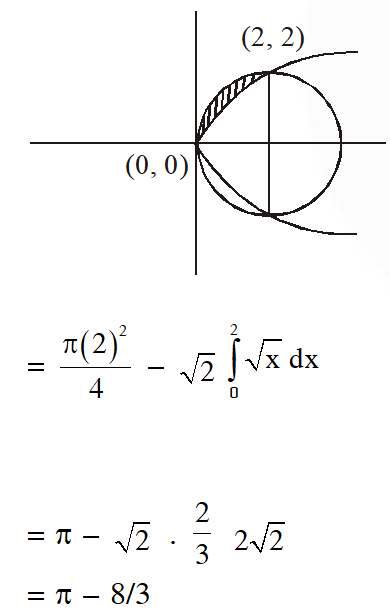Q. The area (in sq. units) of the region $\left\{(\mathrm{x}, \mathrm{y}\}: \mathrm{x} \geq 0, \mathrm{x}+\mathrm{y} \leq 3, \mathrm{x}^{2} \leq 4 \mathrm{y} \text { and } \mathrm{y} \leq 1+\sqrt{\mathrm{x}}\right\}$ is : (1) $\frac{5}{2}$ (2) $\frac{59}{12}$ (3) $\frac{3}{2}$ (4) $\frac{7}{3}$ [JEE(Main)-2017]
Ans. (1)
Q. Let $g(x)=\cos x^{2}, f(x)=\sqrt{x}$ and $\alpha, \beta(\alpha<\beta)$ be the roots of the quadratic equation $18 x^{2}-9 \pi x+\pi^{2}=0 .$ Then the area (in sq. units) bounded by the curve $y=(\operatorname{gof})$ (x) and the lines $x=\alpha, x=\beta$ and $y=0$ is- ( 1)$\frac{1}{2}(\sqrt{3}+1)$ (2) $\frac{1}{2}(\sqrt{3}-\sqrt{2})$ (3) $\frac{1}{2}(\sqrt{2}-1)$ ( 4)$\frac{1}{2}(\sqrt{3}-1)$ [JEE (Main)-2018]
Ans. (4)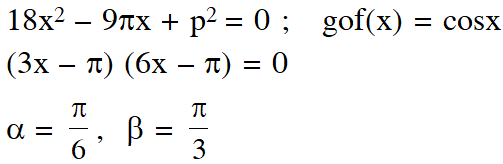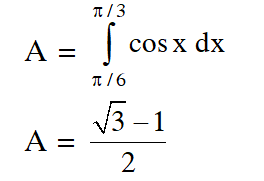mia khalifa
March 23, 2022, 9:36 p.m.
very helpful .... but solution must be needed for better performance
akshat physics wallah
Feb. 23, 2022, 11:05 p.m.
include 2022 Q also
student
May 14, 2021, 8:16 a.m.
really helps revising
D rugved
April 4, 2021, 8:54 p.m.
tq
nikhil
March 11, 2021, 6:55 p.m.
thanku
G.Lakshmi
March 11, 2021, 5:37 p.m.
Nice.....its very useful
Devika mourya
Dec. 18, 2020, 2:51 p.m.